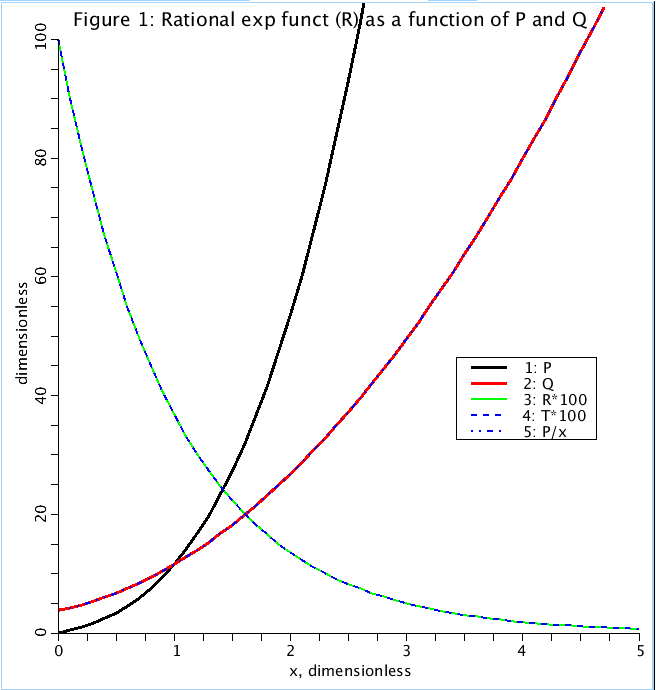# RationalExp

Model number
0421

A rational exponential function, R(x)= exp(-P(x)/Q(x)), where P and Q are polynomial in x expressed p1 + p2*x + p3*x^2 +.. pn*x^(n-1) and 1 + q1*x + q2*x^2 + ....+ qn*x^m, where n is not necessarily = m.

## Description

``` A rational exponential function, R(x) = exp(-P(x)/Q(x)),
where P and Q are polynomial in x expressed p1 + p2*x + p3*x^2 +.. pn*x^(n-1)
and 1 + q1*x + q2*x^2 + ....+ qn*x^m, where n is not necessarily = m.

Such functions are good generalities for monotonic decay curves for many situations:
washout curves, isotopic decays (not alway exponential), pharmacokinetics, or things
rising to a plateau from zero, fitted with F(x) = 1-R(x).

This is a general kind of decay function, the basic form being a single
exponential decay. It can be expanded as desired by adding more terms to P and Q.
With just up to the cubic, the adjustment to the parameters can steepen or make
shallower the inital decay while keeping the tail long.

Consider the TEST (T) curve the standard curve. Note that the Parameter file,
'ConstRloop', run using the 'Loops' page, scales through sets of parameters all
giving the same R curve. With each loop, 1 is added to p1 through p3 and to q0
through q2, but not q3. Explain why this works.

Reducing the curvature: Increasing q2 and q3 does this; increasing q1 causes
slowing of the early downslope but does little to the tail; increasing q2
delays the middle and the tail but not the early downslope.

Increasing the curvature: Increase p1, p2 or p3; put more weight on p3 to bring the
tail down sooner; put weight on p1 and p2 make the initial downslope faster.
```Figure: Rational exponential (R) and four term polynomials P and Q, which make it up, are plotted as a function of x. T is the test exponential of form exp(-x). The coefficients of P and Q are picked so that R fits T.

## Equations

The equations for this model may be viewed by running the JSim model applet and clicking on the Source tab at the bottom left of JSim's Run Time graphical user interface. The equations are written in JSim's Mathematical Modeling Language (MML). See the Introduction to MML and the MML Reference Manual. Additional documentation for MML can be found by using the search option at the Physiome home page.

References

None.

Key terms
compartment
compartmental
decay
first order process
Tutorial
exponential
probability density function
pdf
Acknowledgements

Please cite https://www.imagwiki.nibib.nih.gov/physiome in any publication for which this software is used and send one reprint to the address given below:
The National Simulation Resource, Director J. B. Bassingthwaighte, Department of Bioengineering, University of Washington, Seattle WA 98195-5061.

Model development and archiving support at https://www.imagwiki.nibib.nih.gov/physiome provided by the following grants: NIH U01HL122199 Analyzing the Cardiac Power Grid, 09/15/2015 - 05/31/2020, NIH/NIBIB BE08407 Software Integration, JSim and SBW 6/1/09-5/31/13; NIH/NHLBI T15 HL88516-01 Modeling for Heart, Lung and Blood: From Cell to Organ, 4/1/07-3/31/11; NSF BES-0506477 Adaptive Multi-Scale Model Simulation, 8/15/05-7/31/08; NIH/NHLBI R01 HL073598 Core 3: 3D Imaging and Computer Modeling of the Respiratory Tract, 9/1/04-8/31/09; as well as prior support from NIH/NCRR P41 RR01243 Simulation Resource in Circulatory Mass Transport and Exchange, 12/1/1980-11/30/01 and NIH/NIBIB R01 EB001973 JSim: A Simulation Analysis Platform, 3/1/02-2/28/07.